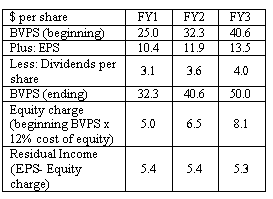### CFA Practice Question

There are 201 practice questions for this study session.

### CFA Practice Question

An analyst is forecasting residual income for a company that has just announced its annual earnings per share of last fiscal year at \$9.2. Analysts expect its earnings to grow at 13.5% a year indefinitely. The company is expected to pay out 30% of its earnings. Its current book value per share is \$25. The cost of equity is 20%. The residual income of the company at the end of the third fiscal year is estimated to be ______.
A. \$5.3
B. \$5.5
C. \$5.6
Explanation: The following table demonstrates calculation of residual earnings for each of the next 3 fiscal years:User Comment
yesficom BVPS FY1= 25*.12 = 3
flape i think 12% is a typo. it has to be 20% and that corresponds with the calc in the chart,
danlan2 BP1=25,
BP2=BP1+9.2*1.135*0.7,
BP3=BP2+9.2*1.135^2*0.7
=25+9.2*1.135*0.7+9.2*1.135^2*0.7
=40.6

NI-BP3*0.2
=9.2*1.135^3-BP3*0.2
=5.33